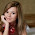# Arithmetic Questions for IBPS PO/Clerk/RBI Astt.

## Arithmetic Questions for IBPS PO/Clerk/RBI Astt.

1. A shopkeeper sold certain items at Rs.630 each, after giving 25% discount on the labelled price. Had he not given the discount, he would have earned a profit of 40% on the cost. What was the cost price of each item?
1) Rs. 600
2) Rs. 540
3) Rs. 800
4) Rs. 720
5) Rs. 750
2. The compound interest on a certain amount for two years is Rs. 1883.52 and the simple interest is Rs. 1728. What is the amount?
1) Rs. 4000
2) Rs. 4500
3) Rs. 4800
4) Rs. 5000
5) Rs. 5400
3. How many numbers between 300 and 1000 can be made with the digits 1, 2, 3, 4, 5, 6, 7 and 0?
1) 280
2) 210
3) 5040
4) 300
5) None of these
4. 15 persons are seated around a table. What is the probability that four particular persons always sit together?
1) 1/546
2) 1/91
3) 4/455
4) 1/2184
5) None of these
5. A person can row 48 km upstream and 70 km downstream in 13 hours and he can row 30 km upstream and 56 km downstream in 9 hours. What is the speed of the person in still water?
1) 4 kmh–1
2) 6 kmh–1
3) 8 kmh–1
4) 10 kmh–1
5) 12 kmh–1
6. Two trains can run at a speed of 30 ms–1 and 25 ms–1 respectively on parallel tracks. When they are running in opposite directions they cross each other in 4 seconds. When they are running in the same direction, a person sitting in the faster train observes that he passes the other train in 28 seconds. What is the ratio of the lengths of the two trains?
1) 5 : 4
2) 7 : 4
3) 5 : 3
4) 7 : 5
5) None of these
7. A man, a woman and a boy can do a work in 40 days, 60 days and 80 days respectively. How many boys must assist 5 men and 6 women to do the work in 4 days?
1) 2
2) 4
3) 5
4) 8
5) 10
8. In a two-digit number the digit in unit’s place is 1.5 times the digit in ten’s place and the number obtained by interchanging the digits is more than the original number by 18. What is 250% of the original number?
1) 115
2) 160
3) 80
4) 70
5) None of these
9. The population of a city increases consistently by 20% per year. If the present population of the city is 151200, what was its population 3 years back?
1) 120000
2) 90000
3) 87500
4) 80000
5) None of these
10. In a cricket match, the captain of one team scored 48 runs more than the average runs scored by the remaining five batsmen of the team who batted in the match. What is the difference between the total runs scored by these five batsmen and the runs scored by the captain?
1) 162
2) 140
3) 132
4) 112

1. 1
2. 3
3. 2
4. 2
5. 4
6. 2
7. 1
8. 1
9. 3
10. 5
###### Share To:### IBTSINDIA.COM

1.